An Ecosystem of δ-Potentials - I An ethereal energy source in Blip 19th July 2020 HOME "Why, for example, do we still not have an international center for climate predictions, which by current estimates would cost “only” \$1 billion spread over 10 years? That’s peanuts compared to what particle physics sucks up, yet vastly more important. Or why, you may have wondered recently, do we not have a center for epidemic modeling? It’s because too much science funding is handed out on the basis of inertia. In the past century, particle physics has grown into a large, very influential and well-connected community. They will keep on building bigger particle colliders as long as they can, simply because that’s what particle physicists do, whether that makes sense or not. It’s about time society takes a more enlightened approach to funding large science projects than continuing to give money to those they have previously given money to. We have bigger problems than measuring the next digit on the mass of the Higgs boson." - Sabine Hossenfelder  in The World Doesn’t Need a New Gigantic Particle Collider, Scientific American, June 19, 2020. *** So far we have developed the idea of a discrete measurement space or j-space, which is solely based on the physical measurements of an infinite source.  Since physical measurements do not allow the precise determination of origin, j-space has to have lower bounds for the measurements of various physical quantities.  Similarly upper bounds must also exist.      In j-space the resource management is very important and different observers have different resources and thus different capacities to perform precise measurements.  The observer Obsc, with measurement capacity (v/c ~1), determines the lower and the upper bounds, based on which the guidelines for the measurements in Aku's universe Blip, are established.      The fabric of j-space consists of j-pixels,  which we have discussed earlier.  In next few blogs we will be discussing, how to introduce physical structures, cosmic and elementary both, in j-space fabric.  We will be using basic quantum mechanics concepts, such as Levinson's theorem,  bound states and  δ-potentials, along with Kruskal-Szekeres coordinates, in j-space. δ-potentials and bound states:      The presence of δ-potentials and subsequent bound states for a particle, is a standard problem in Quantum Mechanics.  The case of a single delta potential and the corresponding bound state for a particle of mass m, is shown below: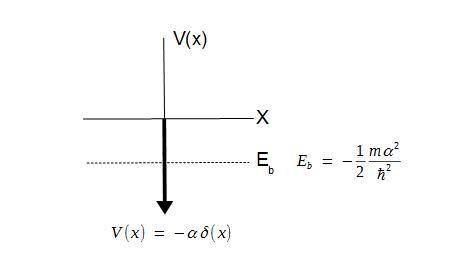The bound state Eb is same as the ground state if the potential is symmetric.   If there are more than one delta functions, there can be more than one bound states depending upon the magnitude of αδ(x).  Our objective in next few blogs, is to discuss the basic conjecture behind the discrete measurement space or j-space, that every object in j-space is in a state of measurement, thus performing a measurement as well as being measured itself. Why do elementary particles exist in j-space, to begin with?     Let us review the expression for the bound state energy in the presence of a delta function: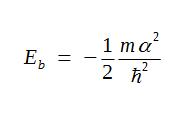The important quantities here are m, α, and ħ.  But first we need to understand what do we mean when we make the statement that "a particle of mass m exists"?     In a measurement space, a measurement is completed when the corresponding circuitis completed i.e. the observer has arrived back at its point of origin.      In the discrete measurement space the objective is to measure the Least-Energy Surface (LES) with the absolute precision, but clearly not all the observers can perform this measurement.  We also note that the physical world exists in region-I of Kruskal-Szekeres coordinates, shown below: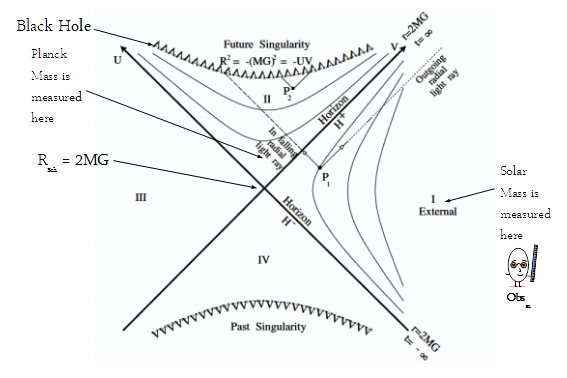The position of the measurement circuit in Kruskal-Szekeres coordinates is around the Event-Horizon Point (EHP), Rsch = 2MG.  In the following diagram various measurement circuits around EHP are shown.  We note that the conventional space and time exist in the external Region-1 only, or in other words a macroscopic observer ObsM, exists in the external Region-I only.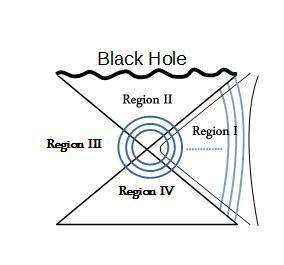The measurement circuits are shown in blue.  The macroscopic observer's capability to make measurements is decreasing as we move from the left to right in the external Region-1, and it is represented by the hyperbolic paths in black.  Sharper the curvature at the vertex, more difficult the measurement, i.e. more and more resources are needed to make a measurement as we move from the right to the left in the external Region-1.      In j-space, the measurement circuit is around LES and the intersection of an hyperbola and an arc of measurement circuit (shown in red) in the external Region-1, represents the measurement of an elementary particle, made by ObsM1.  Thus we have elementary particles corresponding to each red-arc (or equivalently the part of a blue-measurement circuit in Region-I) which potentially can be measured by a macroscopic observer ObsM, given ObsM has sufficient resources to measure it2.  In the following diagram, an observer can measure m2 and m3, but not m1.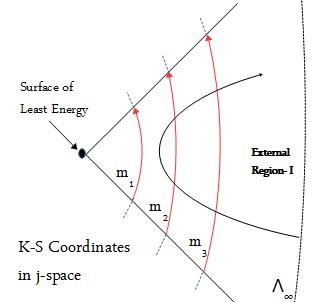To measure the particle m1, the observer making measurement needs to be provided additional resources in the external Region-1.  This situation is shown by the dashed path in the following diagram.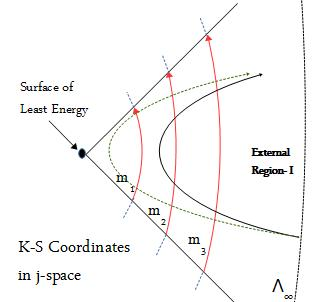This is what particle-colliders are trying to do in a rather rudimentary way.  However it must be clearly understood that no matter how much resources are provided to an observer making measurements in the external region-1, LES can never be measured with an absolute precision.  At best we will have an enormous catalog of particles, afflicted with la maladie exotique, but no new fundamental principle is likely to be discovered.  For that we will have to analyze the results obtained by Astronomy and Cosmology. Nature of bound states in j-space     Let us consider an observer ObsM in j-space, with resources  in the range (Ei, Ef).  Providing a precise value of the energy to an observer in j-space is not possible, as it implies ΔE = 0.  According to uncertainty principle,  ΔEΔt > ħ,  ΔE = 0 means a state with infinite life-time is measured by ObsM.  An infinite life-time measured by ObsM, is finite (∞j) per the measurements of Obsc or Obsi, and hence Δt is always finite and subsequently ΔE is always greater than 0.      The observer ObsM will be measuring the potentials ranging from a shallow-well to a deep-well as shown below: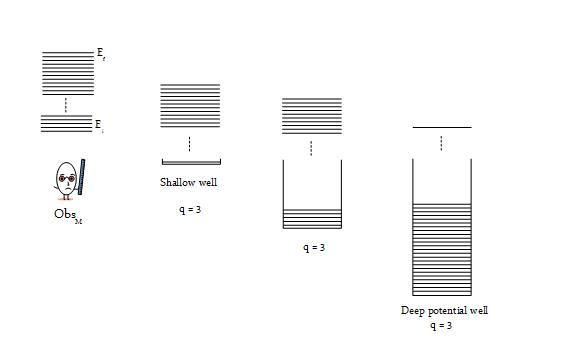We note that as the depth of the potential well increases the observer ObsM has less and less freedom in the free space.          Next we consider an observer Obsc who has infinite, ∞j, resources in j-space.  This observer Obsc will have values for energy ranging from 0j to ∞j, in j-space corresponding to the current information space (q = 3).   We want Obsc to measure a shallow- potential well in a higher information space (q = 2).3 In other words we want Obsc, if possible, to perform a zero entropy measurement in q = 2 information space.     For Obsc since it has ∞j resources, all the potential wells in q = 3 information space, are equivalent to shallow-wells and represent zero-entropy measurements.  However in the case of q = 2 information space, the situation becomes rather interesting.  A shallow-well in q = 2 space is equivalent to δ-potential in q = 3 space even for Obsc.  And δ-potential is, what Obsc is measuring even though Obsc has infinite, ∞j, resources  in q = 3 space, as shown below: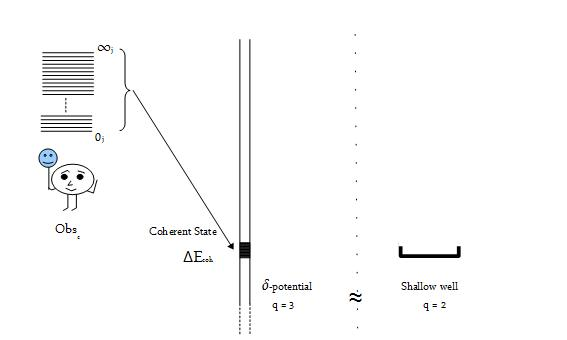In this case, all the states are inside the δ-potential and they form in essence a single bound state or a "coherent" state of the width ΔE ~ 0.  Thus the nature of the bound state in q = 3 measurement space, while measuring a shallow well from q = 2 space, is that of a coherent state Ecoh.  The measurement of δ-potential in Blip     Our interest is in understanding how the measurements of the coherent state Ecoh, manifest themselves into physical parameters measured in Blip.  Blip is represented by the measurements made by the macroscopic observer ObsM,  in the external region-I in K-S coordinates.      Since the energy of the coherent state Ecoh in q = 3 information space, has a minimum limit ∞j, the corresponding rest mass mo = E × (c2)-1, will be infinite or extremely heavy, even though the physical dimensions may be in Planck's domain, in the q = 3 space or equivalently in the external region-I of KS Coordinates.     The coherent state will have a minimum spread ΔEcoh ~ 0j, per ObsM measurements.  The uncertainty principle correlates ΔEcoh with the coherent state life-time tcoh in external region-I, as ΔEcoh × tcoh ~ ħ.  Since ΔEcoh is very small, the coherent state life-time tcoh will be exceeding large (~∞j).       Next, in external Region-I, an infinitesimal change in entropy of the coherent state dScoh and the change in the energy to bring about the infinitesimal change dScoh in the entropy dEcoh,  are correlated to the temperature Tcoh of the coherent state as:It will take infinite amount of resources (dEcoh ~ ∞), to bring about infinitesimal change in the entropy (dScoh ~ 0), of the coherent state under discussion.  Hence the temperature of the coherent state as measured by the macroscopic observer ObsM, will be exceedingly high. Finally an infinite energy-source in Blip     Therefore in the discrete measurement space, the shallow-well from a higher-information-space|q=2 is equivalent to a δ-potential in the current-information-space|q=3.  The information corresponding to the shallow-well|q=2, will be measured as a coherent-state|q=3 by a macroscopic observer ObsM, in Blip (equivalently q=3 information state or the external region-I of KS coordinates).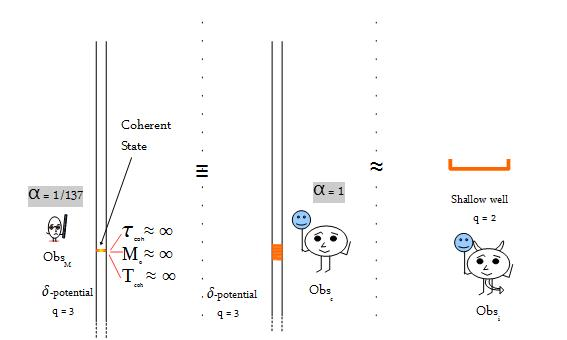This coherent state will result in an extremely massive entity in Blip, with an infinite life-time and infinite temperature.  The infiniteness of the coherent state Ecoh, disappears as Ecoh slowly radiates away, in the external Region-I (in a few billion years probably).    Lastly, the "measured-mass" and the temperature of the coherent state, as they are determined in Blip, are statistical quantities inversely related to the spread in the energy ΔEcoh, of the coherent state|q=3, similar to the life-time tcoh. ____________________ 1. The physical world of Blip, exists at Λ-plane. 2.  Action in j-space is defined as the resources provided to an observer traveling the path PQ, at the point P, such that the observer can arrive at the point Q unassisted.  In external region-I of KS coordinates, action translates into the physical variables, energy E or the momentum k.  3. Different information spaces do not agree upon the definitions of Lorentz and Möbius invariances. *** To be continued.. Previous Blogs: EPR Paradox-I  Nutshell-2015Information on www.ijspace.org is licensed under a Creative Commons Attribution 4.0 International License. Attribution — You must give appropriate credit, provide a link to the license, and indicate if changes were made. You may do so in any reasonable manner, but not in any way that suggests the licensor endorses you or your use. No additional restrictions — You may not apply legal terms or technological measures that legally restrict others from doing anything the license permits. This is a human-readable summary of (and not a substitute for) the license.